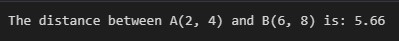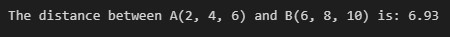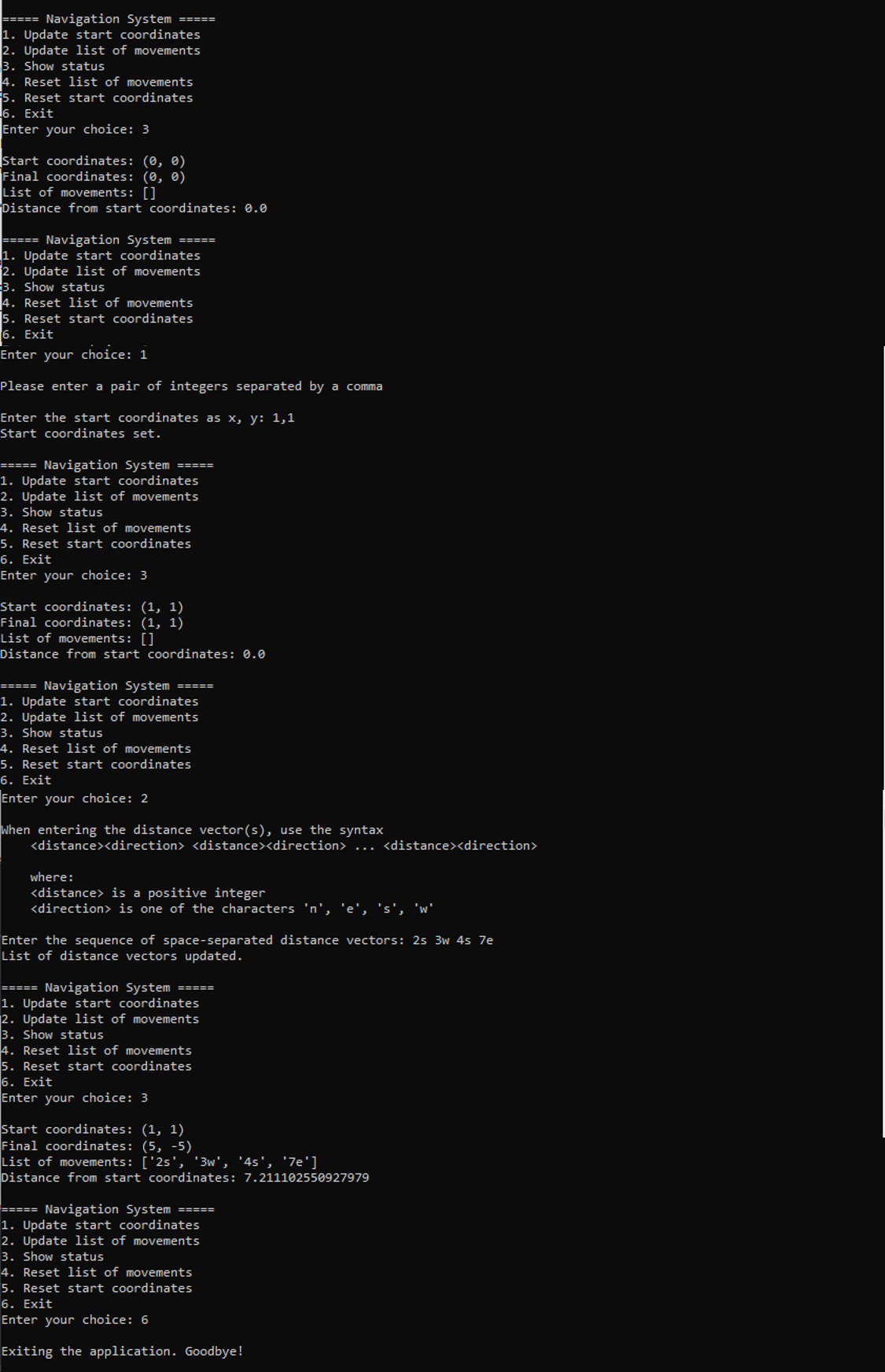## Python Math Dist | How to use math.dist function?

##### Aniekan

Distance calculations play a crucial role in various fields, from navigation systems to scientific research.

In order to ease the calculations involved in distance computation. Python provides a versatile function in the  `math` module; the `dist` function. This function makes it easier to carry out distance calculations between two points in two, three, or more dimensions.

In this article, we will embark on an exploration of the use of this function, and a simple application that illustrates how the `math.dist` function may be used in real life.

Let’s dive in!

## Using `math.dist()` function

Before we can make use of the `math.dist()` function, we need to import the `math` module, one of Python’s inbuilt modules. This module provides a wide range of math functions and constants, like trigonometric, logarithmic functions, exponential functions, and so on. Since the `math` module comes with Python, and therefore, we do not need a separate installation of the module. So our code will begin with:

``````import math
``````

In order to calculate the distance between two points, we need to supply the points as tuples to `dist`(though a list can still be used to represent each point). The following code fragment shows the use of the `dist` function to calculate the Euclidean distance between the two points:

``````import math

# Coordinates of points A and B
A = 2, 4
B  = 6, 8

# Calculating the distance using math.dist()
distance = round(math.dist(A, B), 2)

print(f"The distance between A{A} and B{B} is: {distance}")
``````

In this code snippet, we have two points, A(2, 4) and B(6, 8), in a 2D space. We then passed the two points,  represented by the tuples `A` and `B` , into `math.dist()`, which then returned the result below;If we were to do this using the Euclidean distance, our code will look like the code listed below:

``````import math

# Coordinates of points A and B
A = 2, 4
B  = 6, 8

# Calculating the distance using the Euclidean distance formula
distance = round(math.sqrt((B - A)**2 + (B - A)**2), 2)

print(f"The distance between A{A} and B{B} is: {distance}")
``````

This code looks more involved. Happily, `math.dist` does all the work for us!

If we wanted to still do things ourselves, but in this case, we made use of the Euclidean formula to calculate the distance between points in 3D space, our code could look like the following:

``````import math

# Coordinates of points A and B
A = 2, 4, 6
B = 6, 8, 10

# Calculating the distance using the Euclidean distance formula
distance = round(math.sqrt((B - A)**2 + (B - A)**2 + (B - A)**2), 2)

print(f"The distance between A{A} and B{B} is: {distance}")
``````

Our code is more involved than the 2D code! The output looks as follows:If we do it ourselves, our code gets more and more complex as the dimension of the points gets higher. Also, we need a different code for each dimension. However, Python saves us work by automating everything for us in the `math.dist` function. Yes, you heard right! `math.dist` can also handle the above calculation without complicating our code. The code, using `math.dist`, would look as follows:

``````import math

# Coordinates of points A and B
A = 2, 4, 6
B = 6, 8, 10

# Calculating the distance using math.dist()
distance = round(math.dist(A, B), 2)

print(f"The distance between A{A} and B{B} is: {distance}")
``````

The output will be the same as the one we got with the Euclidean formula for the 3D points we used earlier. Now that we have seen how to make use of `math.dist`, we now want to create a simple navigation application that will help us calculate distance.

## Example Application of `math.dist`: Navigation System

Imagine that we are travelling on foot from a start position, and we are allowed to move in the directions North, East, South, and West. We might need a navigation system that will help us carry out the following:

• Get the final 2D coordinates of our journey
• Give us the distance from our home to our current position

We will create a simple Python console application that will help us with this. Our application will allow us to:

• Enter the start coordinates.
• Update the list of movements we have carried out so far.
• Show the status, that is, the start and final coordinates, the movements made, and the distance moved from the start coordinate to get to the final coordinate.
• Reset the list of movements.
• Reset the start coordinates to 0, 0
• Exit the application

### Main script: Initialization

Our main script starts with the initialization of `start_coord` (representing the starting coordinates. Initially set to `(0, 0)`), and `parsed_distances` , representing the parsed distance vectors entered by the user, is initialized to an empty list.

``````start_coord = 0, 0
parsed_distances = []
``````

### Main Script: Main Loop

The main loop is responsible for presenting the user with a menu of options continually until the user chooses to exit the application.

``````while True:
print("1. Update start coordinates")
print("2. Update list of movements")
print("3. Show status")
print("4. Reset list of movements")
print("5. Reset start coordinates")
print("6. Exit")

choice = input("Enter your choice: ")

if choice == '1':
pass

elif choice == '2':
pass

elif choice == '3':
pass

elif choice == '4':
pass

elif choice == '5':
pass

elif choice == '6':
pass

else:
pass
``````

Within the main loop, the menu options are displayed for the user to choose from. Whatever the user enters is saved to the `choice` variable.

#### Menu Option 1: Update start coordinates

If the user chooses option 1 (to enter start coordinates), we have the following code snippet to handle the user selection:

``````if choice == '1':
print("\nPlease enter a pair of integers separated by a comma\n")
response = input("Enter the start coordinates as x, y: ")
try:
parsed_response = [int(coord.strip()) for coord in response.split(",")]

if len(parsed_response)!=2:
raise ValueError()

except:
print("Invalid value(s) for start coordinates")

else:
start_coord = tuple(parsed_response)
print("Start coordinates set.")
``````

The user will be prompted to enter a pair of integers separated by a comma (e.g., `x, y`) to set the start coordinates. If the user enters the correct input, the `start_coord` variable is updated with the parsed coordinates.

#### Menu option 2: Update the list of movements

If option 2 was selected, it means the user needs to update the list of movements he/she has made so far. The code snippet is shown below:

``````elif choice == '2':
print("\nWhen entering the distance vector(s), use the syntax")
print("    <distance><direction> <distance><direction>   ...
<distance><direction> \n")
print("    where:")
print("    [distance] is a positive integer")
print("    [direction] is one of the characters 'n', 'e', 's', 'w'\n")

user_input = input("Enter the sequence of space-separated distance vectors: ")
distances = user_input.split()  # Split the input into individual distances

try:
parsed_distances += parse_distances(distances)  # Parse the distances with error handling
print("List of distance vectors updated.")

except ValueError as e:
print("Error:", str(e))
``````

The user is prompted to input a sequence of space-separated distance vectors. Recall that the allowed directions are North, East, South, and West. So, the user will need to enter, for example

`12e 3n 2s 2w 7n`

Representing a movement of `12m` to the East, then `3m` to the North, then `2m` to the South, then `2m` to the West, and finally, `7m` to the North. The input from the user is then parsed by the function `parse_distances`in order to check for incorrect input from the user, and then added to the `parsed_distances` list.

The code for the function `parse_distances` is shown below:

``````def parse_distances(input_distances):
parsed_distances = []

for i in range(len(input_distances)):
distance = input_distances[i]
direction = distance[-1].lower()  # Get the direction (last character) and convert to lowercase

try:
value = int(distance[:-1])  # Get the numerical value (excluding the last character)

except:
raise ValueError(f"Invalid distance value @ index {i}: " + distance)

else:
# Check for negative values or invalid directions
if value < 0 or direction not in ['e', 'w', 'n', 's']:
raise ValueError(f"Invalid distance @ index {i}: " + distance)

parsed_distances.append((value, direction))

return parsed_distances``````

The function takes the distances entered by the user as input and parses them into a list of tuples. Each tuple is a pair of the form (distance travelled, direction moved). The distance travelled is a scalar (a non-negative integer), while the direction is one out of the following items `n`, `e`, `s`, `w` (representing the allowed directions the user can travel).

If there is a problem with the user input, an exception is raised by the function. Otherwise, a list is created containing the valid distance vectors (as a list of tuples), and returned to the caller.

#### Menu Option 3: Show application status

Option 3 displays the current application status to the user. When this is selected, the code will display the start coordinates, final coordinates, list of movements, and distance from the start coordinates. the code to do this is shown below:

``````elif choice == '3':
# Calculate the distance and coordinates
final_distance, end_coord = calculate_distance(parsed_distances, start_coord)
print("\nStart coordinates:", start_coord)
print("Final coordinates:", end_coord)
print("List of movements:", [str(d)+d for d in parsed_distances])
print("Distance from start coordinates:", final_distance)
``````

The distance and coordinates are calculated using the `calculate_distance()` function. The current values of the `parsed_distances` and `start_coord` variables are passed as arguments to the function.

`calculate_distance()` Carries out the distance and final coordinate calculation. the code for the function is shown below:

``````def calculate_distance(parsed_distances, start_coord):
start_x, start_y = start_coord
end_x, end_y = start_x, start_y

for distance in parsed_distances:
value, direction = distance

if direction == 'e':
end_x += value
elif direction == 'w':
end_x -= value
elif direction == 'n':
end_y += value
elif direction == 's':
end_y -= value

final_distance = math.dist((start_x, start_y), (end_x, end_y))  # Calculate the Euclidean distance between start and end coordinates

return final_distance, (end_x, end_y)
``````

The function takes the `parsed_distances` list and the `start_coord` tuple as input. In order to simplify the distance vectors in `parsed_distances`, it iterates over the list and updates the end x or y coordinates depending on the direction specified for each distance.

Finally, the function makes use of `math.dist` to calculate the Euclidean distance between the start and end coordinates, and returns the final distance and the end coordinates together as a tuple.

#### Menu Options 4 and 5: Reset start coordinates and list of movements

Options 4 and 5 are reset options for the user: 4 allows the user to reset the list of movements, while 5 resets the start coordinates. See the code snippet below for how this is implemented:

``````elif choice == '4':
parsed_distances = []
print("\nList of movements reset.")

elif choice == '5':
start_coord = 0, 0
print("\nStart coordinates reset.")``````

From the code snippet, we can see that rest was rather straightforward; The `parsed_distances` list is cleared of all distances, while `start_coord` is set back to (0, 0).

#### Menu Option 6: Exit application

Finally, if the user selected Exit, (the 6th option), a farewell message will be displayed, and the loop will be exited (via the `break` statement) effectively exiting the program. See the code fragment below for the implementation:

``````elif choice == '6':
print("\nExiting the application. Goodbye!")
break
``````

The complete code listing is shown below:

``````import math

# Function to parse the distance input and handle errors
def parse_distances(input_distances):
parsed_distances = []

for i in range(len(input_distances)):
distance = input_distances[i]
direction = distance[-1].lower()  # Get the direction (last character) and convert to lowercase

try:
value = int(distance[:-1])  # Get the numerical value (excluding the last character)

except:
raise ValueError(f"Invalid distance value @ index {i}: " + distance)

else:
# Check for negative values or invalid directions
if value < 0 or direction not in ['e', 'w', 'n', 's']:
raise ValueError(f"Invalid distance @ index {i}: " + distance)

parsed_distances.append((value, direction))

return parsed_distances

# Function to calculate the final distance and coordinates
def calculate_distance(parsed_distances, start_coord):
start_x, start_y = start_coord
end_x, end_y = start_x, start_y

for distance in parsed_distances:
value, direction = distance

if direction == 'e':
end_x += value
elif direction == 'w':
end_x -= value
elif direction == 'n':
end_y += value
elif direction == 's':
end_y -= value

final_distance = math.dist((start_x, start_y), (end_x, end_y))  # Calculate the Euclidean distance between start and end coordinates

return final_distance, (end_x, end_y)

# Main code
start_coord = 0, 0
parsed_distances = []

while True:
print("1. Update start coordinates")
print("2. Update list of movements")
print("3. Show status")
print("4. Reset list of movements")
print("5. Reset start coordinates")
print("6. Exit")

choice = input("Enter your choice: ")

if choice == '1':
print("\nPlease enter a pair of integers separated by a comma\n")
response = input("Enter the start coordinates as x, y: ")
try:
parsed_response = [int(coord.strip()) for coord in response.split(",")]

if len(parsed_response)!=2:
raise ValueError()

except:
print("Invalid value(s) for start coordinates")

else:
start_coord = tuple(parsed_response)
print("Start coordinates set.")

elif choice == '2':
print("\nWhen entering the distance vector(s), use the syntax")
print("      ... \n")
print("    where:")
print("     is a positive integer")
print("     is one of the characters 'n', 'e', 's', 'w'\n")

user_input = input("Enter the sequence of space-separated distance vectors: ")
distances = user_input.split()  # Split the input into individual distances

try:
parsed_distances += parse_distances(distances)  # Parse the distances with error handling
print("List of distance vectors updated.")

except ValueError as e:
print("Error:", str(e))

elif choice == '3':
# Calculate the distance and coordinates
final_distance, end_coord = calculate_distance(parsed_distances, start_coord)
print("\nStart coordinates:", start_coord)
print("Final coordinates:", end_coord)
print("List of movements:", [str(d)+d for d in parsed_distances])
print("Distance from start coordinates:", final_distance)

elif choice == '4':
parsed_distances = []
print("\nList of movements reset.")

elif choice == '5':
start_coord = 0, 0
print("\nStart coordinates reset.")

elif choice == '6':
print("\nExiting the application. Goodbye!")
break

else:

``````

When this our code is executed, we get the following example session:We have explored the use of `math.dist` in calculating the Euclidean distance between two points. We’ve explored how to calculate the distance in both 2D and 3D spaces, and then we implemented a simple navigation system that made use of `math.dist` to calculate the distance a traveller has covered from a start point.

Armed with the `math.dist` function, when we can make distance calculations without having to modify our code because the dimension of the start and destination points have changed.